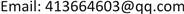CSAComputer Science and Application2161-8801Scientific Research Publishing10.12677/CSA.2019.91005CSA-28436CSA20190100000_41841224.pdf信息通讯 融合用户微博兴趣挖掘与协同过滤的新闻推荐 News Recommendation Combining User Microblog Interests Mining and Collaborative Filtering 21平华21靖宇21广东工业大学计算机学院，广东 广州null0801201909013845© Copyright 2014 by authors and Scientific Research Publishing Inc. 2014This work is licensed under the Creative Commons Attribution International License (CC BY). http://creativecommons.org/licenses/by/4.0/Copyright © 2019 by authors and Hans Publishers Inc.

This work is licensed under the Creative Commons Attribution International License (CC BY).

http://creativecommons.org/licenses/by/4.0/1. 引言

2. 推荐框架

1) 兴趣挖掘模块。用户微博中的数据有如文本、图片、视频、标签、关注者和粉丝等各种数据。本模块主要针对文本、标签、关注者、转发和评论数据进行挖掘，用来构建用户兴趣集进行新闻推荐。

2) 推荐模块。本模块将经过分类和关键词提取处理后的新闻与用户微博兴趣集计算匹配相似的新闻。通过计算新闻集合用户兴趣集的相似度并结合协同过滤来获得推荐列表，并将推荐列表进行因素调整排序进行推荐，最后验证推荐效果。

3. 兴趣挖掘模块

U f b = { ( t 1 , w 1 ) , ( t 2 , w 2 ) , ⋯ , ( t n , w n ) }

U F = ∑ U ∈ ( U B , U M , U f b ) U (1)

U p = { m , p , n , t } (2)

U F P = ( 1 − α ) U F + α U p , α ∈ [ 0 , 1 ] (3)

4. 推荐模块4.1. 新闻分类

4.2. 新闻推荐

W i j = [ n u m ( t i j ) t o t a l ( D i ) ] * log [ N n i j ] (4)

D ( i ) = [ − ( t p − t n ) 2 t B + 1 ] (5)

W i j = [ n u m ( t i j ) t o t a l ( D i ) ] * log [ N n i j ] * D ( i ) (6)

s i m ( U F P , j ) = ∑ w i , w j = 1 k W I j * W i j (7)

U s e r S i m ( U , V ) = U N ∩ V N U N ∪ V N (8)

∑ I = 0 n U s e r S i m ( U , V I ) = ∑ I = 0 n V I N − U N ∩ V I N (9)

E X P ( U , V I , I j ) = τ ∑ j = 0 m S i m ( U ( U ∈ U F P ) , I j ) + ζ ∑ I = 0 n U s e r S i m ( U , V I ) (10)

5. 实验与分析5.1. 数据集

5.2. 实验

p r e c i s i o n = R N ( L ) L (11)

r e c a l l = R N ( L ) R ( L ) (12)

F 1 = 2 × p r e c i s i o n × r e c a l l p r e c i s i o n + r e c a l l (13)

5.3. 实验对比算法

1) 基于内容的推荐(CB)：该算法的主要思想是根据用户以往历史浏览新闻纪录，将历史浏览新闻作为用户兴趣集，推荐给用户相似的新闻。

2) 基于用户的推荐(UCF)：通过找到具有相似兴趣的邻居用户，将邻居用户其他的阅览新闻推荐给用户。算法如式(9)。

5.4. 实验结果与分析

6. 总结